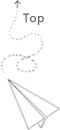# Model-approach

### Physical model

The simple thing is the tellurion or the DNA model or the molecure model. Or you can assume the planet is a point with the weight in the solar system or universe.

The physical model in computer is the current trend. The fancy name is the digital twin.

Easy to modify and test, with less cost.

The core part of the physical model is to abstract the key idea from the the object we research on.

Another kind of physical model is to abstract the workflow, such as using Gant graph or the pipeline. It visulizes how things move forward in a way that is easy to analyze

### Mathmetical model

The input is the a bunch of data, we use mathmetical model to represent these data sets and to show how these data are generated.

According to the amount of data we can use different techniques. If there are limited number of data, we may use the time series processing or all kinds of curve fitting model. If there are large amount of data such as several gigabytes figures, we may use the deep learning model to process it. Anyway, the core idea is to find the distribution behind the limited sample data points.

### Combine them together

The good project or research always need to combine these two models together. When we have a new problem, consider the solution from these two perspectives is always a good start.

## 推荐文章